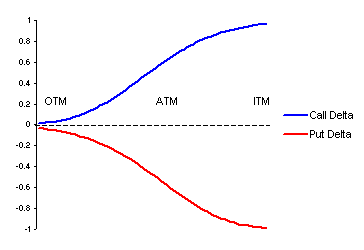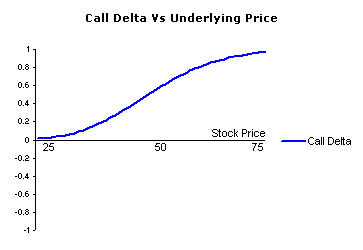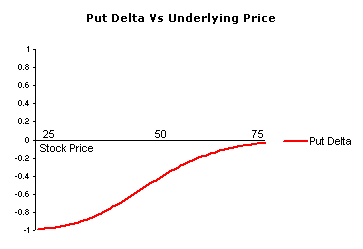## Thursday, November 22, 2007

### Option Delta

The delta of an option is the sensitivity of an option price relative to changes in the price of the underlying asset. It tells option traders how fast the price of the option will change as the underlying stock/future moves.The above graph illustrates the behaviour of both call and put option deltas as they shift from being out-of-the-money (OTM) to at-the-money (ATM) and finally in-the-money (ITM). Note that calls and puts have opposite deltas - call options are positive and put options are negative.

Option delta is represented as the price change given a 1 point move in the underlying asset and is usually displayed as a decimal value. Delta values range between 0 and 1 for call options and -1 to 0 for put options. Note - some traders refer to the delta as a whole number between 0 to 100 for call options and -100 to 0 for put options. However, I will use the decimal version of -1 to 0 (puts) and 0 to 1 (calls) throughout this site.

## Call Options

Whenever you are long a call option, your delta will always be a positive number between 0 and 1. When the underlying stock or futures contract increases in price, the value of your call option will also increase by the call options delta value. Conversely, when the underlying market price decreases the value of your call option will also decrease by the amount of the delta.The above graph shows how the delta of a call option changes as the underlying price changes.

When the call option is deep in-the-money and has a delta of 1, then the call will move point for point in the same direction as movements in the underlying asset.

## Put Options

Put options have negative deltas, which will range between -1 and 0. When the underlying market price increases the value of your put option will decreases by the amount of the delta value. Conversely, when the price of the underlying asset decreases, the value of the put option will increase by the amount of the delta value.The above graph shows how the delta of a put option changes as the underlying price changes. So, when the underlying price rallies, the price of the option will decrease by delta amount and the put delta will also decrease as the option moves further out-of-the-money.

Source : http://www.optiontradingtips.com